Disclaimer: This is an example of a student written essay.

Any scientific information contained within this essay should not be treated as fact, this content is to be used for educational purposes only and may contain factual inaccuracies or be out of date.

# Non Equillibrium Green’s Function Technique

 ✅ Paper Type: Free Essay ✅ Subject: Physics ✅ Wordcount: 4774 words ✅ Published: 12th Mar 2018

NON EQUILLIBRIUM GREEN’S FUNCTION TECHNIQUE USED FOR THE METAL-INSULATOR-METAL DIODES

ANSHUMAN

Electronics and Communication Dept. NIT Kurukshetra

Abstract – In this paper theoretical analysis of NEGF method, including the transport equation and Poisson equation, is done followed by the derivation of an analytical model using NEGF tunnelling probability through any number of insulating layers. Numerical NEGF simulator are shown matching with the AF-TMM simulator results.

1. INTRODUCTION

THE STUDY OF tunnelling phenomena in Metal Insulator Metal (MIM) is an important topic for the aim of the development of rectennas for energy harvesting and infrared detectors applications. Although the interest in Metal-Insulator-Metal (MIM) diodes dates back to 1950s –, but they attracted the attention again in the last few years due to its applications, energy harvesting – and infrared/terahertz detectors –.

Earlier, various analytical expressions for the tunnelling transmission probability through MIM diodes were developed based on WKB approximation –. However, the WKB does not take into consideration the wave function reflections at the interface between different layers . Therefore, there came the need for other models to simulate the tunnelling probability. Non Equilibrium Green Function (NEGF)  numerical method is one of the methods used to calculate the tunnelling transmission probability –. It is an accurate numerical method, but it needs long time of calculations on a PC in comparison to other analytical models.

Any program used for the simulation of a device performs a solution of transport equation and “Poisson” equation .The transport equation gives the electron density, n(r) and the current, I for a known potential profile U(r), while Poisson equation gives the effective U(r), felt by an electron due to the presence of other electron in its vicinity.

Here, in this paper the Quantum transport, Green’s functions and its various equations under non equilibrium condition are discussed and a detailed quantum mechanical modeling of the tunnelling current through MIM diodes is presented. An analytical expression for the tunnelling transmission probability is presented using the NEGF equations for any number of insulator layers between the two metals.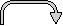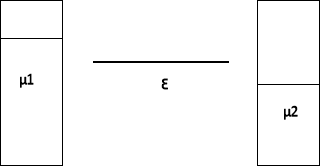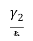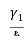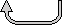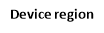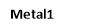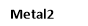Fig.1. Transport of electrons for single energy level device

The paper is organized as follows: in Section II, the transport equations are discussed. In section III NEGF equations for MIM Diode is described in detail. The governing equations and numerical implementation of it is outlined. The material parameters used in the simulation are also summarized.

1. GENERAL TRANSPORT EQUATION

Let’s consider the model for a single device sandwiched between two metals 1and 2ION THE METAL-INSULATOR.ce of other electron in its vicinity.port and “111111111111111111111111111111111111111111, as shown in fig. 1.The device is assumed to be having a single energy level, Ô. Our first aim is to find the number of electrons, N in the device. Let Ef be the Fermi level set by the work function of the two metal contacts under the equilibrium condition. On applying the bias voltage, Vb between metal 1 and 2, the Fermi- energies of two metals gets modified to µ1 and µ2 respectively and given as :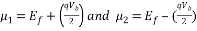(1)

This difference in Fermi-energy gives rise to a non-equilibrium condition and hence two different Fermi-functions for the two contacts. If device is in equilibrium with metal 1, then number of electrons will be f­1 but if it is in equilibrium with metal 2, number of electrons will be f­2, where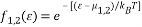(2)

Let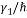and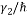be the rate of escape of electron from device into metal 1 and metal 2 respectively. Therefore the currents I1 and I2 crossing metal1 and 2 interfaces are given as: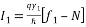And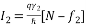(3)

For I1 = I2 = I, we get steady-state number of electrons N and current I as: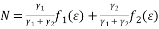(4a)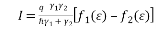(4b)

Due to the applied bias voltage one of the reservoir keeps pumping the electron trying to increase the number while the other keeps emptying it trying to lower the number. Ultimately, there is a continuous flow of current, I (eq. 4b) in the external circuit.

Assuming ðœ‡1 > ðœ€ > μ2 and the temperature is low enough that f1 (ε) ≡ f0 (εμ1) ≈ 1 and f2 (ε) ≡ f0 (εμ2) ≈ 0, the Eq. 4b simplifies to :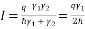If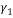=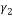(5)

Eq.5 suggests that we can flow an unlimited current through this one level device if we increase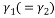, i.e. by coupling the device more and strongly to the metal contacts. But the maximum conductance of a one-level device is equal to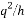, so there must be some reduction factor.

This reduction is due to the broadening of the discrete level that occurs because of increased coupling of the device with the two metals. This broadened discrete level can be described by the distribution: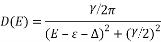With line-width of γ and shift of level from ε to ε+âˆ†, where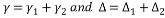. This broadening phenomena modifies the Eqs. (4a, b) to include an integral over all energies weighted by the distribution D(E) :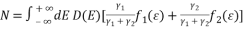(6a)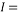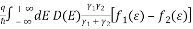(6b)

Using algebraic manipulation Eqs. (6a, b) becomes: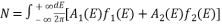(7a)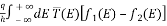(7b)

Where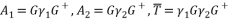(8)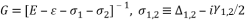(9)

Till now we have discussed device with single energy level ε. But in practical situation (i.e. for real devices) there exist multiple energy levels. Any device, in general, can be represented by a Hamiltonian matrix, whose eigenvalues tells about the allowed energy levels. For example if we describe the device using an effective mass Hamiltonian H =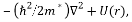then it can be represented with a (NxN) matrix by choosing a discrete lattice with N point and applying methods of finite-differences . This corresponds to using a discretized real space basis.

Similarly, we define self-energy matrices [∑­1,2] which describe the broadening and shift of energy levels due to coupling with the two metals. The required NEGF equations now can be obtained from Eqs(7a, b) by replacing scalar quantities ε and σ­1,2 with the corresponding matrices [H] and [∑­1,2], and is given as: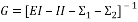,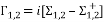(10)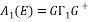,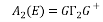(11)

The number of electrons N, in the device is replaced with the density matrix, given by: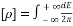{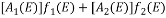} (12)

Current is still represented by Eq. (7b). The transmission can be given as the trace of the analogous matrix quantity: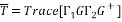(13)

1. TRANSMISSION EQUATION FOR MIM DIODE USING NEGF EQUATIONS

The 1D time-independent single-particle Schrödinger equation is given by :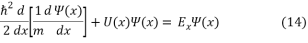Where,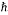is the reduced Plank constant, ψ(x) is the electron wave-function, m is the effective mass and U(x) is the potential energy.

If it is assumed that the insulator layers are divided into M grid points having uniform spacing, a, then finite difference discretization on the 1D grid is applied to Schrödinger equation Eq. (1) at each node i as follows :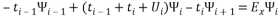(2)

Where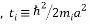, represents the interaction between the nearest neighbour grid points i and i+1, UiU (xi), and mi is the electron effective mass between the nodes i and i + 1. The coupling of the potential barrier to the left and right metal electrodes is taken into consideration by rewriting Eq. (1) for i =1 and i = M with open boundary conditions expressed at Metal1/Insulator and Insulator/Metal2 interfaces. So, Schrödinger equation now takes the following form :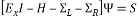(3)

Where, H is the M × M Hamiltonian matrix of the insulator potential, I is the M × M identity matrix, ψ is the wavefunction M × 1 vector and S is M × 1 vector. L and R are the M × M self-energies of the left and right contacts respectively.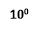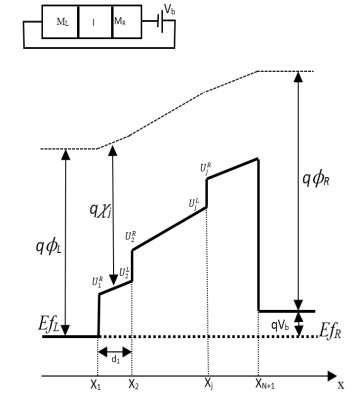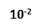Fig. 1. Potential of a stack of N insulator materials under applied bias voltage, Vb. Each insulator layer is characterized by a barrier height (Uj), a thickness (d j), a dielectric constant ε j, and an effective mass (m j).

Now, under a tri-diagonal form H can be rewritten as: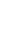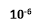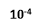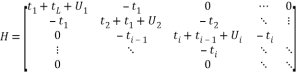L and R are given as: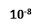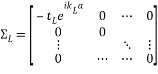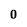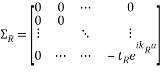The solution of Eq. (1) can be given in the terms of retarded Green’s function as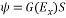where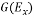is M×M retarded Green’s function :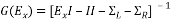The rate of escape of electron to either left or right metal from a given state can be taken into consideration by defining two quantities, ΓL and ΓR .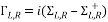Hence, the tunnelling probability can now be computed as :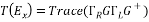1. COMPARISION OF NEGF MODEL WITH OTHER MODELS

A model of MIIM diode was simulated using NEGF, AF-TMM and WKB Approximation for a comparative analysis of their transmission probability vs. electron transmission energy curve. The parameter spacing, a, for the NEGF calculation was assumed equal to the hundredth of the insulator layer thickness. This was found adequate for reasonable simulation time. The effective mass was assumed equal to the free mass of the electron throughout the MIM structure.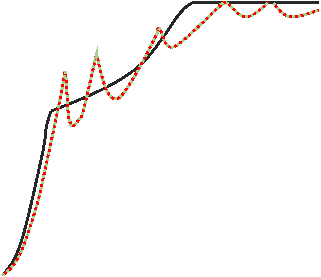Fig.3 below shows the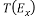of the simulated MIIM of Nb/Nb2O5 (2nm)-Ta2O5 (1nm)/Nb at 0.1V of bias voltage. A complete matching between AF-TMM and NEGF results is observed.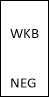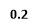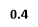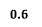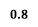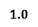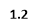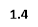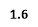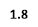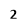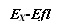Fig.3. Transmission probability T (Ex) versus the electron transmission energy calculated using AF-TMM, NEGF, and WKB at Vb = 0.1 V for Nb/Nb2O5/Ta2O5/ Nb MIIM diode.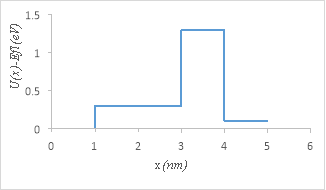Fig.4. Energy band diagram of the MIIM diode used for simulation

REFERENCES

1. J. G. Simmons, “Electric tunnel effect between dissimilar electrodes separated by a thin insulating film,” J. Appl. Phys., vol. 34, no. 9, pp. 2581–2590, Mar. 1963.
2. J. G. Simmons, “Generalized formula for the electric tunnel effect between similar electrodes separated by a thin insulating film,” J. Appl. Phys., vol. 34, no. 6, pp. 1793–1803, 1963.
3. R. Stratton, “Volt-current characteristics for tunneling through insulating films,” J. Phys. Chem. Solids, vol. 23, no. 9, pp. 1177–1190, Sep. 1962.
4. J. G. Simmons, “Potential barriers and emission-limited current flow between closely spaced parallel metal electrodes,” J. Appl. Phys., vol. 35, no. 8, pp. 2472–2481, Aug. 1964.
5. S. Grover and G. Moddel, “Applicability of metal/insulator/metal (MIM) diodes to solar rectennas,” IEEE J. Photovoltaic, vol. 1, no. 1, pp. 78–83, Jul. 2011.
6. K. Choi, M. Dagenais, F. Yesilkoy, A. N. Chryssis, and M. C. Peckerar, “Solar spectrum rectification using Nano-antenna and tunneling diodes,” Proc. SPIE, vol. 7605, pp. 1–12, Feb. 2010.
7. M. R. Osgood, B. R. Kimball, and J. Carlson, “Nanoantenna-coupled MIM nanodiodes for efficient vis/nir energy conversion,” Proc. SPIE, vol. 6652, no. 1, pp. 1–11, Sep. 2007.
8. R. Osgood, J. B. Carlson, B. R. Kimball, D. P. Ziegler, J. R. Welch, L. E. Belton, G. E. Fernandes, Z. Liu, and J. Xu “Plasmonic nanorectennas for energy conversion,” Proc. SPIE, vol. 7394, pp. 1–11, Aug. 2009.
9. J. A. Bean, A. Weeks, and G. D. Boreman, “Performance optimization of antenna-coupled tunnel diode infrared detectors,” IEEE J. Quantum Electron., vol. 47, no. 1, pp. 126–135, Jan. 2011.
10. B. Tiwari, J. A. Bean, G. Szakmany, G. H. Bernstein, P. Fay, and W. Porod, “Controlled etching and regrowth of tunnel oxide for antenna coupled metal-oxide-metal diodes,” J. Vacuum Sci. Technol. B, Microelectron. Nanometer Struct. , vol. 27, no. 5, pp. 2153–2160, 2009.
11. J. A. Bean, B. Tiwari, G. H. Bernstein, P. Fay, and W. Porod, “Thermal infrared detection using dipole antenna-coupled metal-oxide-metal diodes,” J. Vacuum Sci. Technol. B, Microelectron. Nanometer Struct. , vol. 27, no. 1, pp. 11–14, 2009.
12. Arun Goud Akkala, ”NEGF Simulation of electron transport in resonant inteband tunneling diodes”, Purdue University, pp. 8-18, 2011.
13. Supriyo Dutta, “Nanoscale device modeling: the Green’s function method,” Superlattices and Microstructures, vol. 28, no. 4, pp.253-272, 2000.
14. Islam E. Hashem, Nadia H. Rafat and Ezzaldin A. Soliman, “Theoretical study of metal-insulator-metal tunneling diode figure of merit,” IEEE Journal of Quantum Electronics, vol. 9, no. 1, pp. 72-80, January 2013
15. M. Moreau, D. Munteanu, and J.-L. Autran, “Simulation of gate tunneling current in metal–insulator–metal capacitor with multilayer high-κ dielectric stack using the non-equilibrium green’s function formalism,” Jpn. J. Appl. Phys., vol. 48, no. 11, pp. 111409-1–111409-8, Nov. 2009.
16. D. Munteanu, J. L. Autran, M. Moreau, and M. Houssa, “Electron transport through high-κ dielectric barriers: A non-equilibrium Green’s function (NEGF) study,” J. Non-Crystall. Sol., vol. 355, no. 21, pp. 1180–1184, Jul. 2009.
17. S. Grover and G. Moddel, “Engineering the current-voltage characteristics of metal-insulator-metal diodes using double-insulator tunnel barriers,” Solid-State Electron., vol. 67, no. 1, pp. 94–99, Jan. 2012.
18. A. K. Ghatak, K. Thyagarajan, and M. R. Shenoy, “A novel numerical technique for solving the one-dimensional Schrödinger equation using matrix approach-application to quantum well structures,” IEEE J. Quantum Electron., vol. 24, no. 8, pp. 1524–1531, Aug. 1988.
19. Supriyo Dutta, “The Non-equilibrium green’s function (NEGF) formalism: An elementary introduction,” IEEE IEDM, pp. 703-706, 2002.
20. Supriyo Dutta, Quantum transport: atom to transistor, 1st ed., Cambridge University Press, 2005, pp.1-30

SYMBOLS

 Symbol Units q electronic charge 1.602 × 10−19 C H Planck constant 6.626 × 10−34 J s â„ h/2π 1.055 × 10−34 J s M free electron mass 9.11 × 10−31 kg ðœº0 permittivity of free space 8.854 × 10−12 F/m q2/h Conductance quantum 38.7 × 10−6 S t0 â„ 2/2m*a2 electron-volts (eV) N number of electrons or number of photons dimensionless ð† density matrix dimensionless ε energy level eV H Hamiltonian eV U self-consistent potential eV E energy eV μ electrochemical potential eV f(E) Fermi function dimensionless D(E) density of states (DOS) /eV A(E) spectral function /eV G(E) Green’s function (retarded) /eV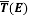transmission function dimensionless T (E) transmission probability (<1) dimensionless ðœ¸ ,ðšª(E) broadening eV ðšº(E) self-energy (retarded) eV

View all

## DMCA / Removal Request

If you are the original writer of this essay and no longer wish to have your work published on UKEssays.com then please:

Related Services

Prices from

BHD57

Approximate costs for:

• 1000 words
• 7 day deliveryHumanity University

Dedicated to your worth and value as a human being!

Related Lectures